# IBDP Maths AI: Topic: SL 4.2: Presentation of data: IB style Questions SL Paper 1

## Question

The table below shows the number of words in the extended essays of an IB class.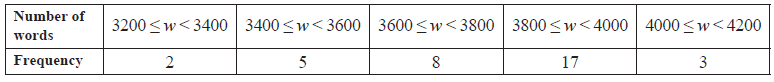Draw a histogram on the grid below for the data in this table.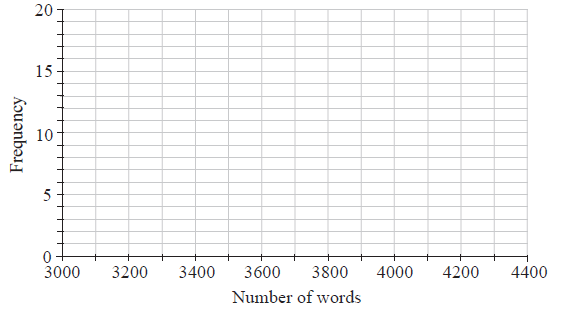a.

Write down the modal group.

b.

The maximum word count is $$4000$$ words.

Write down the probability that a student chosen at random is on or over the word count.

c.

## Markscheme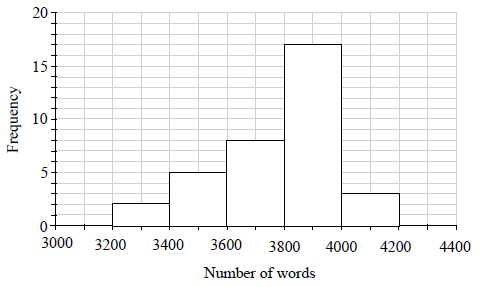(A3)     (C3)

Notes: (A3) for correct histogram, (A2) for one error, (A1) for two errors, (A0) for more than two errors.
Award maximum (A2) if lines do not appear to be drawn with a ruler.
Award maximum (A2) if a frequency polygon is drawn.[3 marks]

a.

$${\text{Modal group}} = 3800 \leqslant w < 4000$$     (A1)     (C1)[1 mark]

b.

$${\text{Probability}} = \frac{3}{{35}}{\text{ }}(0.0857{\text{, }}8.57\% )$$     (A1)(A1)     (C2)

Note: (A1) for correct numerator (A1) for correct denominator.[2 marks]

c.

## Question

The following histogram shows the weights of a number of frozen chickens in a supermarket. The weights are grouped such that $$1 \leqslant {\text{weight}} < 2$$, $$2 \leqslant {\text{weight}} < 3$$ and so on.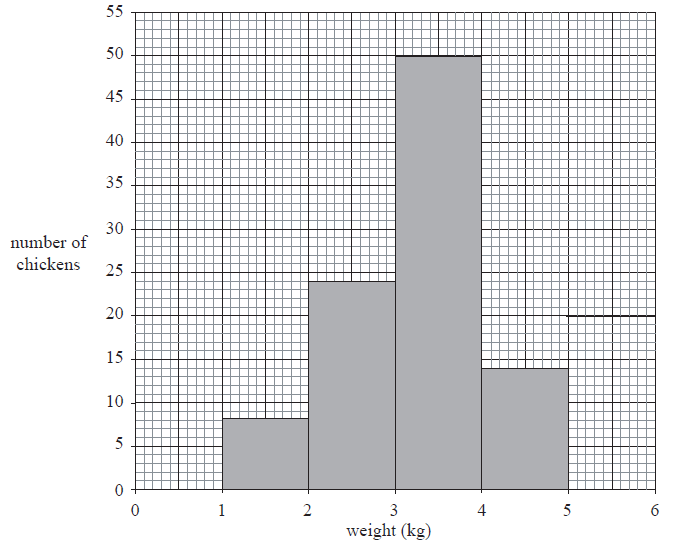Find the total number of chickens.

b.

Write down the modal group.

c.

Gabriel chooses a chicken at random.

Find the probability that this chicken weighs less than $$4{\text{ kg}}$$.

d.

## Markscheme

$$96$$     (A1)     (C1)[1 mark]

b.

$$3 \leqslant {\text{weight}} < 4{\text{ kg}}$$ . Accept $$3 – 4{\text{ kg}}$$     (A1)     (C1)[1 mark]

c.

For adding three heights or subtracting $$14$$ from $$96$$     (M1)

$$\frac{{82}}{{96}}{\text{ }}(0.854{\text{ or }}\frac{{41}}{{48}}{\text{, }}85.4\% )$$ (ft) from (b).     (A1)(ft)     (C2)[2 marks]

d.

## Question

The distribution of the weights, correct to the nearest kilogram, of the members of a football club is shown in the following table.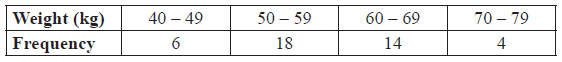On the grid below draw a histogram to show the above weight distribution.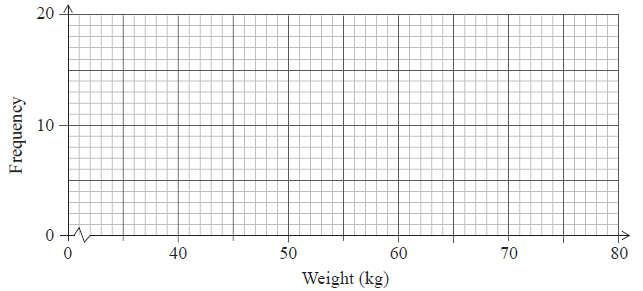a.

Write down the mid-interval value for the $$40 – 49$$ interval.

b.

Find an estimate of the mean weight of the members of the club.

c.

Write down an estimate of the standard deviation of their weights.

d.

## Markscheme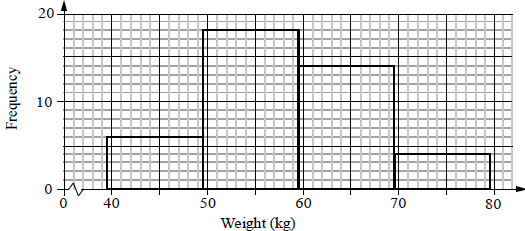(A1)(A1)     (C2)

Notes: (A1) for all correct heights, (A1) for all correct end points ($$39.5$$, $$49.5$$ etc.).

Histogram must be drawn with a ruler (straight edge) and endpoints must be clear.
Award (A1) only if both correct histogram and correct frequency polygon drawn. [2 marks]

a.

$$44.5$$     (A1)     (C1)

Note: If (b) is given as $$45$$ then award
(b) $$45$$     (A0)
(c) $$58.8{\text{ kg}}$$     (M1)(A1)(ft) or (C2)(ft) if no working seen.
(d) $$8.44$$     (C1)

[1 mark]

b.

Unit penalty (UP) applies in this question.

$${\text{Mean}} = \frac{{44.5 \times 6 + 54.5 \times 18 + \ldots }}{{42}}$$     (M1)

Note: (M1) for a sum of frequencies multiplied by midpoint values divided by $$42$$.

$$= 58.3{\text{ kg}}$$     (A1)(ft)     (C2)

Note: Award (A1)(A0)(AP) for $$58$$.

Note:
If (b) is given as $$45$$ then award

(b) $$45$$     (A0)
(c) $$58.8{\text{ kg}}$$     (M1)(A1)(ft) or (C2)(ft) if no working seen.
(d) $$8.44$$     (C1)[2 marks]

c.

$${\text{Standard deviation}} = 8.44$$     (A1)     (C1)

Note: If (b) is given as $$45$$ then award
(b) $$45$$     (A0)
(c) $$58.8{\text{ kg}}$$     (M1)(A1)(ft) or (C2)(ft) if no working seen.
(d) $$8.44$$     (C1)[1 mark]

d.

## Question

120 Mathematics students in a school sat an examination. Their scores (given as a percentage) were summarized on a cumulative frequency diagram. This diagram is given below.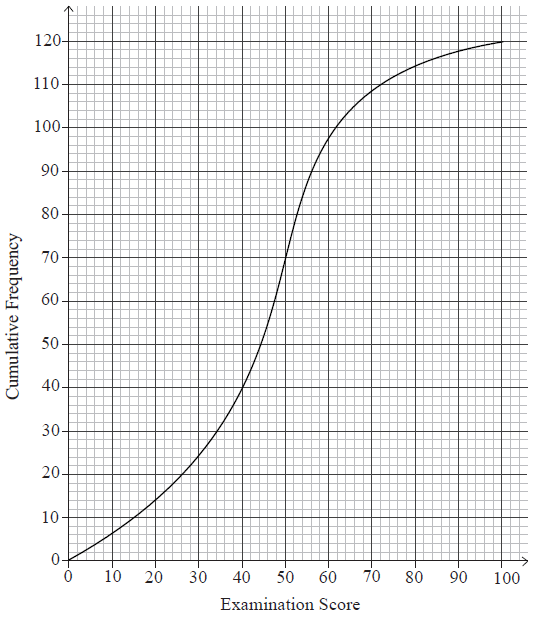Complete the grouped frequency table for the students.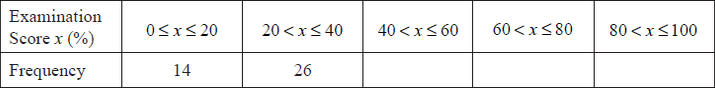a.

Write down the mid-interval value of the $$40 < x \leqslant 60$$ interval.

b.

Calculate an estimate of the mean examination score of the students.

c.

## Markscheme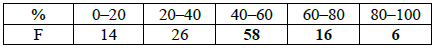(A1)(A1)(A1)     (C3)[3 marks]

a.

50     (A1)    (C1)[1 mark]

b.

$${\text{Mean}} = \frac{{10 \times 14 + ……. + 90 \times 6}}{{120}}$$     (M1)

Note: Award (M1) for correct substitution of their values from (a) in mean formula.

$$= 45\frac{2}{3}(45.7)$$     (A1)(ft)     (C2)[2 marks]

c.

## Question

The weights of 90 students in a school were recorded. The information is displayed in the following table.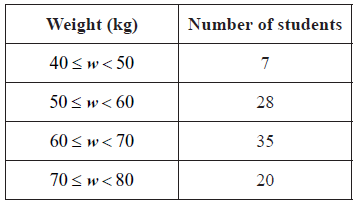Write down the mid interval value for the interval $$50 \leqslant w \leqslant 60$$.

a.

Use your graphic display calculator to find an estimate for the mean weight.

b.i.

Use your graphic display calculator to find an estimate for the standard deviation.

b.ii.

Find the weight that is 3 standard deviations below the mean.

c.

## Markscheme

55     (A1)     (C1)[1 mark]

a.

$$62.\bar 5{\text{ }}\left( {62.6} \right)$$     (A2)(ft)     (C2)[2 marks]

b.i.

8.86     (A1)     (C1)

b.ii.

62.6 – 3 × 8.86 = 36.0     (M1)(A1)(ft)     (C2)

Note: Accept 36.

Follow through from their values in part (b) only if working is seen.[2 marks]

c.

## Question

Toronto’s annual snowfall, x, in cm, has been recorded for the past 176 years. The results are shown in the table.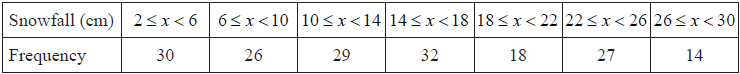Write down the modal class.

a.

Write down the mid interval value for the class 6 ≤ x < 10 .

b.

Calculate an estimate of the mean annual snowfall.

c.

Find the number of years for which the annual snowfall was at least 18 cm.

d.

## Markscheme

14 ≤ x < 18     (A1)     (C1)[1 mark]

a.

8     (A1)     (C1)[1 mark]

b.

$$\frac{{{\text{4}} \times {\text{30 + 8}} \times {\text{26 + 12}} \times {\text{29 + 16}} \times {\text{32 + 20}} \times {\text{18 + 24}} \times {\text{27 + 28}} \times {\text{14}}}}{{176}}$$     (M1)

Notes: Award (M1) for an attempt to substitute their mid-interval values (consistent with their answer to part (b)) into the formula for the mean. Award (M1) where a table is constructed with their (consistent) mid-interval values listed along with the frequencies.

= 14.7 (cm) (14.7045…)     (A1)(ft)     (C2)

Notes: Follow through from their answer to part (b). If a final incorrect answer that is consistent with their (b) is given award (M1)(A1)(ft) even if no working is seen.[2 marks]

c.

18 + 27 + 14     (M1)

Note: Award (M1) for adding 18, 27 and 14.

= 59     (A1)     (C2)[2 marks]

d.

## Question

The cumulative frequency graph represents the speed, s, in $${\text{km }}{{\text{h}}^{ – 1}}$$, of 80 cars passing a speed camera.Write down the number of cars passing the camera with speed of less than or equal to 50 $${\text{km}}\,{{\text{h}}^{ – 1}}$$.

a.

Complete the following grouped frequency table for $$s$$, the speed of the cars passing the camera.b.

Write down the mid-interval value of the $$50 < s \leqslant 70$$ interval.

c.

Use your graphic display calculator to find an estimate of

(i)     the mean speed of the cars passing the camera;

(ii)     the standard deviation of the speed of the cars passing the camera.

d.

## Markscheme

10     (A1)     (C1)[1 mark]

a.(A1)(ft)     (C1)

b.

60     (A1)     (C1)[1 mark]

c.

(i)     $$67.5 \left( {{\text{km}}\,{{\text{h}}^{ – 1}}} \right)$$     (A2)(ft)

Notes: Award (M1) for an attempt to use the formula for the mean with at least two midpoint values consistent with their answer to part (c). Follow through from their table in part (b).

(ii)     $$18.6 (18.6413…)$$     (A1)(ft)     (C3)

Note: Follow through from their table in part (b).[3 marks]

d.

## Question

A group of students were asked how long they spend practising mathematics during the week. The results are shown in the following table.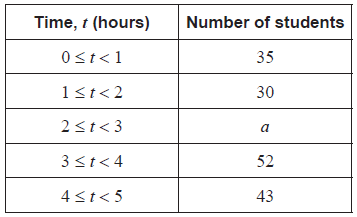It is known that $$35 < a < 52$$ .

Write down

i)      the modal class;

ii)     the mid-interval value of the modal class;

iii)    the class in which the median lies.

a.

For this group of students, the estimated mean number of hours spent practising mathematics is $$2.69$$.

Calculate the value of $$a$$ .

b.

## Markscheme

i)      $$3 \leqslant t < 4$$        (A1)    (C1)

Note: Accept equivalent notation: $$[3,\,\,4)$$ or $$[3,\,\,4[$$.

ii)     $$3.5$$        (A1)(ft)    (C1)

Note: Follow through from part (a)(i).

iii)    $$2 \leqslant t < 3$$        (A1)(ft)    (C1)

Note: Follow through from part (a)(i), for consistent misuse of inequality. Accept equivalent notation: $$[2,\,\,3)$$ or $$[2,\,\,3[$$.

a.

$$\frac{{3.5 \times 0.5 + 30 \times 1.5 + a \times 2.5 + 52 \times 3.5 + 43 \times 4.5}}{{35 + 30 + a + 52 + 43}} = 2.69$$        (M1)(A1)(ft)

Notes: Award (M1) for substitution into mean formula and equating to $$2.69$$, (A1)(ft) for correct substitutions. Follow through from their mid-interval value in part (a)(ii).

$$(a = )\,\,40$$       (A1)(ft)     (C3)

Note: The final (A1)(ft) is awarded only if $$a$$ is an integer and $$35 < a < 52$$. Follow through from part (a)(ii).

b.

## Question

The lengths of trout in a fisherman’s catch were recorded over one month, and are represented in the following histogram.Complete the following table.a.

State whether length of trout is a continuous or discrete variable.

b.

Write down the modal class.

c.

Any trout with length 40 cm or less is returned to the lake.

Calculate the percentage of the fisherman’s catch that is returned to the lake.

d.

## Markscheme(A2)     (C2)

Note:     Award (A2) for all correct entries, (A1) for 3 correct entries.[2 marks]

a.

continuous     (A1)     (C1)[1 mark]

b.

$${\text{60 (cm)}} < {\text{trout length}} \leqslant {\text{70 (cm)}}$$     (A1)     (C1)

Note:     Accept equivalent notation such as $$(60,{\text{ }}70]$$ or $$]60,{\text{ }}70]$$.

Award (A0) for “60-70” (incorrect notation).[1 mark]

c.

$$\frac{4}{{22}} \times 100$$     (M1)

Note:     Award (M1) for their 4 divided by their 22.

$$= 18.2{\text{ }}(18.1818 \ldots )$$     (A1)(ft)     (C2)

Note:     Follow through from their part (a). Do not accept 0.181818….[2 marks]

d.

## Question

In a high school, 160 students completed a questionnaire which asked for the number of people they are following on a social media website. The results were recorded in the following box-and-whisker diagram.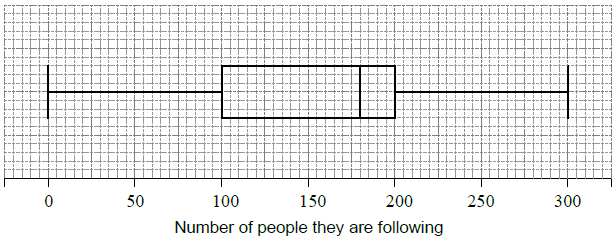The following incomplete table shows the distribution of the responses from these 160 students.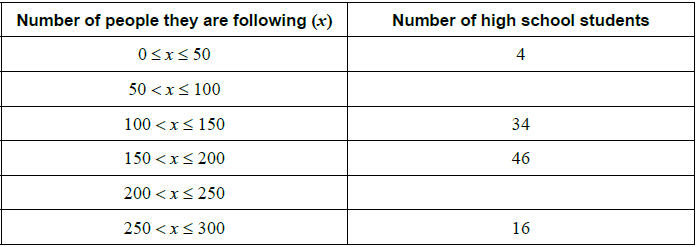Write down the median.

a.

Complete the table.

b.

Write down the mid-interval value for the 100 < x ≤ 150 group.

c.i.

Using the table, calculate an estimate for the mean number of people being followed on the social media website by these 160 students.

c.ii.

## Markscheme

180   (A1) (C1)[1 mark]

a.

36, 24     (A1)(A1) (C2)

Note: Award (A0)(A1) for two incorrect values that add up to 60.[2 marks]

b.

125 (accept 125.5)     (A1)

c.i.

$$\frac{{4 \times 25 + 36 \times 75 + 34 \times 125 + 46 \times 175 + 24 \times 225 + 16 \times 275}}{{160}}$$     (M1)

Note: Award (M1) for correct substitution of their mid-interval values, multiplied by their frequencies, into mean formula.

=156 (155.625)     (A1)(ft) (C3)

Note: Follow through from parts (b) and (c)(i).[3 marks]

c.ii.

## Question

The histogram shows the time, t, in minutes, that it takes the customers of a restaurant to eat their lunch on one particular day. Each customer took less than 25 minutes.

The histogram is incomplete, and only shows data for 0 ≤ t < 20.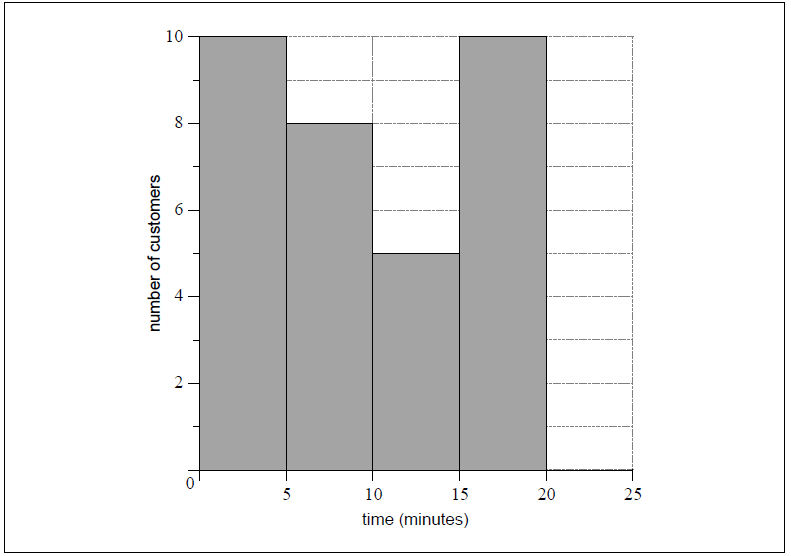The mean time it took all customers to eat their lunch was estimated to be 12 minutes.

It was found that k customers took between 20 and 25 minutes to eat their lunch.

Write down the mid-interval value for 10 ≤ t < 15.

a.

Write down the total number of customers in terms of k.

b.i.

Calculate the value of k.

b.ii.

Hence, complete the histogram.

c.

## Markscheme

12.5     (A1) (C1)[1 mark]

a.

33 + k  OR  10 + 8 + 5 + 10 + k     (A1)

Note: Award (A1) for “number of customers = 33 + k”.[1 mark]

b.i.

$$\frac{{2.5 \times 10 + 7.5 \times 8 + \ldots + 22.5 \times k}}{{33 + k}} = 12$$     (M1)(A1)(ft)

Note: Award (M1) for substitution into the mean formula and equating to 12, (A1)(ft) for their correct substitutions.

(k =) 7     (A1)(ft) (C4)

Note: Follow through from part (b)(i) and their mid-interval values, consistent with part (a). Do not award final (A1) if answer is not an integer.[3 marks]

b.ii.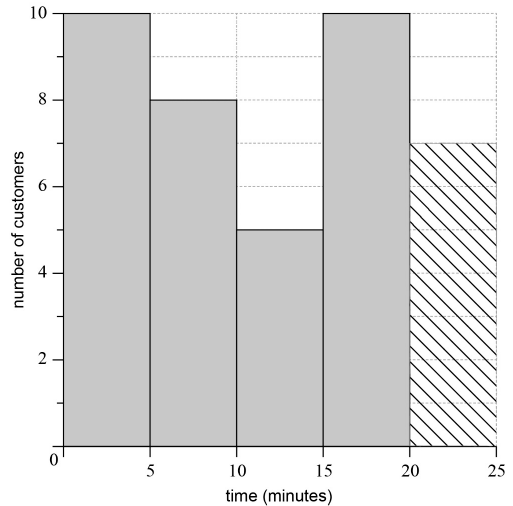(A1)(ft) (C1)

Note: Follow through from their part (b)(ii) but only if the value is between 1 and 10, inclusive.[1 mark]

c.

## Question

Consider the frequency histogram for the distribution of the time, $$t$$ , in minutes of telephone calls that Helen made last week.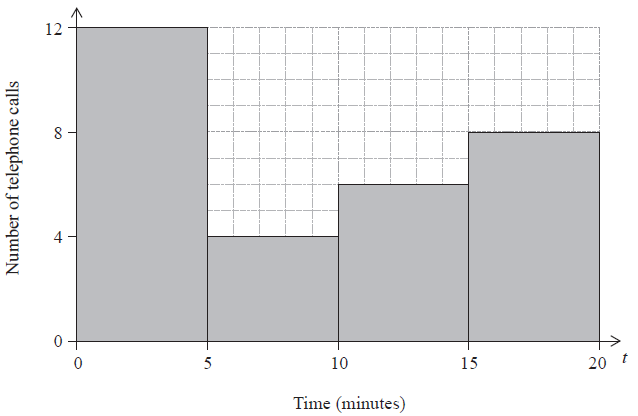Complete the frequency table for this distribution.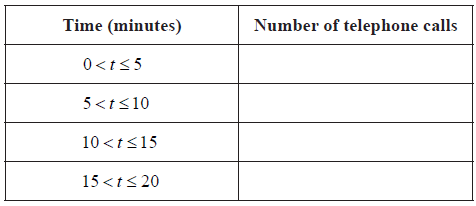a.

Write down the modal class.

b.

Write down the mid interval value of the $$10 < t \leqslant 15$$ class.

c.

Use your graphic display calculator to find an estimate for the mean time.

d.

## Markscheme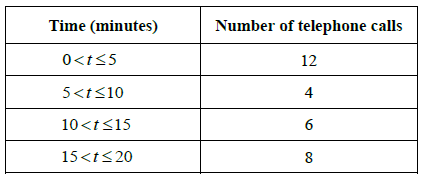(A2)     (C2)

Note: Award (A2) for four correct entries, (A1) for three correct entries, (A0) otherwise.

a.

$$0 < t \leqslant 5$$     (A1)     (C1)

b.

$$12.5$$     (A1)     (C1)

c.

$$\frac{{275}}{{30}}$$     (M1)

Note: Award (M1) for division with $$275$$ seen.

$$= 9.17$$ ($$9.16666 \ldots$$)     (A1)(ft)     (C2)

Note: Follow through from their parts (a) and (c), irrespective of whether working is shown.

d.

## Question

The grades obtained by a group of $$20$$ IB students are listed below: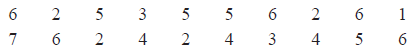Complete the following table for the grades obtained by the students.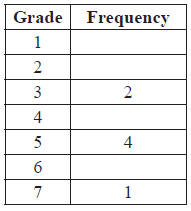a.

Write down the modal grade obtained by the students.

b.

Calculate the median grade obtained by the students.

c.

One student is chosen at random from the group.

Find the probability that this student obtained either grade $$4$$ or grade $$5$$.

d.

## Markscheme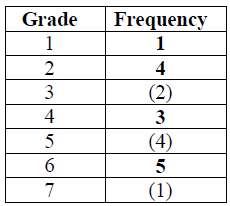(A2)     (C2)

Notes: Award (A1) for three correct. Award (A0) for two or fewer correct.[2 marks]

a.

$${\text{Mode}} = 6$$     (A1)(ft)     (C1)[1 mark]

b.

$${\text{Median}} = 4.5$$     (M1)(A1)(ft)     (C2)

Note: (M1) for attempt to order raw data (if frequency table not used) or (M1) halfway between 10th and 11th result.[2 marks]

c.

$$\frac{7}{{20}}{\text{ }}(0.35{\text{, }}35\% )$$     (A1)(ft)     (C1)[1 mark]

d.

## Question

$$80$$ matches were played in a football tournament. The following table shows the number of goals scored in all matches.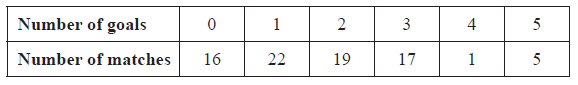Find the mean number of goals scored per match.

a.

Find the median number of goals scored per match.

b.

A local newspaper claims that the mean number of goals scored per match is two. Calculate the percentage error in the local newspaper’s claim.

c.

## Markscheme

$$\frac{{0 \times 16 + 1 \times 22 + 2 \times 19 \ldots }}{{80}}$$     (M1)

Note: Award (M1) for substituting correct values into mean formula.

1.75     (A1)     (C2)[2 marks]

a.

An attempt to enumerate the number of goals scored.     (M1)

$$2$$     (A1)     (C2)[2 marks]

b.

$$\frac{{2 – 1.75}}{{1.75}} \times 100$$     (M1)
$$14.3 \%$$     (A1)(ft)     (C2)

Notes: Award (M1) for correctly substituted $$\%$$ error formula. $$\%$$ sign not required. Follow through from their answer to part (a). If $$100$$ is missing and answer incorrect award (M0)(A0). If $$100$$ is missing and answer incorrectly rounded award (M1)(A1)(ft)(AP).[2 marks]

c.

## Question

The table shows the number of bicycles owned by 50 households.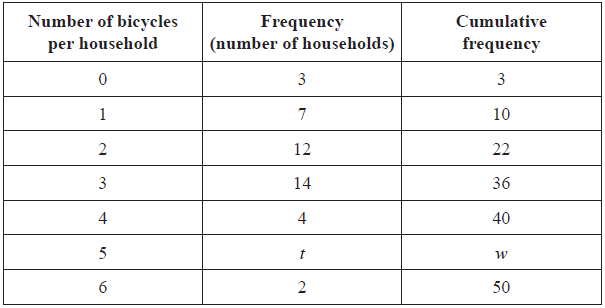Write down the value of

(i) t ;

(ii) w .

a.

Indicate with a tick (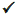) whether the following statements are True or False.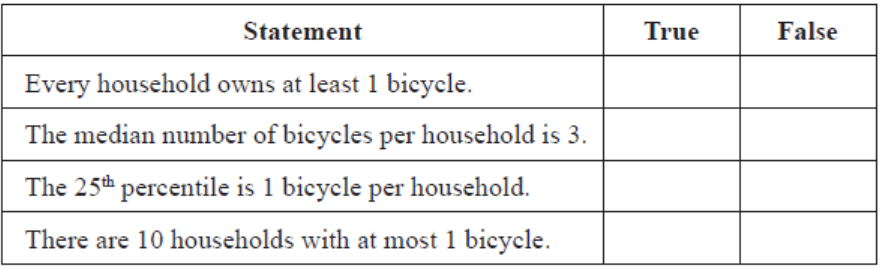b.

## Markscheme

(i) 8     (A1)

(ii) 48     (A1)(ft)     (C2)

Note: Follow through from their t, even if no workings seen as long as w < 50.

a.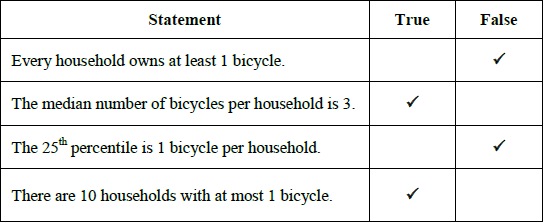(A1)(A1)(A1)(A1)     (C4)

b.

## Question

In a particular week, the number of eggs laid by each hen on a farm was counted. The results are summarized in the following table.State whether these data are discrete or continuous.

a.

Write down

(i)     the number of hens on the farm;

(ii)     the modal number of eggs laid.

b.

Calculate

(i)     the mean number of eggs laid;

(ii)     the standard deviation.

c.

## Markscheme

discrete     (A1)     (C1)

a.

(i)     60     (A1)

(ii)     5     (A1)     (C2)

b.

(i)     $$\frac{{1 \times 4 + 2 \times 7 + 3 \times 12 \ldots }}{{60}}$$     (M1)

Notes: Award (M1) for an attempt to substitute into the “mean of a set of data” formula, with at least three correct terms in the numerator.

Denominator must be 60.

Follow through from part (b)(i), only if work is seen.

$$= 4.03{\text{ }}(4.03333 \ldots )$$     (A1)

Notes: Award at most (M1)(A0) for an answer of 4 but only if working seen.

(ii)     $$1.54{\text{ }}(1.53803 \ldots )$$     (A1)     (C3)

c.

## Question

Two groups of 40 students were asked how many books they have read in the last two months. The results for the first group are shown in the following table.The quartiles for these results are 3 and 5.

Write down the value of the median for these results.

a.

Draw a box-and-whisker diagram for these results on the following grid.b.

The results for the second group of 40 students are shown in the following box-and-whisker diagram.Estimate the number of students in the second group who have read at least 6 books.

c.

## Markscheme

$$4$$     (A1)(C1)

a.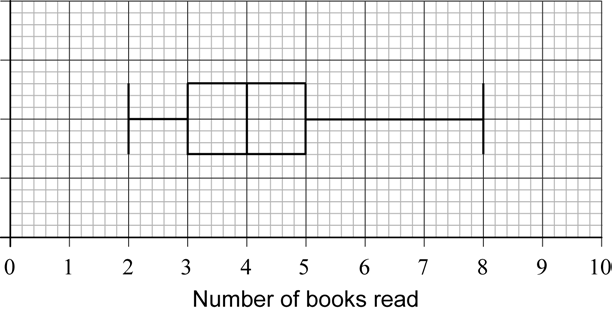(A1)(ft)(A1)(A1)     (C3)

Notes: Award (A1)(ft) for correct median, (A1) for correct quartiles and box, (A1) for endpoints 2 and 8 joined by a straight line that does not cross the box. Follow through from their median from part (a).

b.

$$40 \times 0.25$$     (M1)

Notes: Award (M1) for $$40 \times 25\% \;\;\;$$OR$$\;\;\;40 – 40 \times 75\%$$.

$$10$$     (A1)     (C2)

c.

## Question

The lengths of trout in a fisherman’s catch were recorded over one month, and are represented in the following histogram.Complete the following table.a.

State whether length of trout is a continuous or discrete variable.

b.

Write down the modal class.

c.

Any trout with length 40 cm or less is returned to the lake.

Calculate the percentage of the fisherman’s catch that is returned to the lake.

d.

## Markscheme(A2)     (C2)

Note:     Award (A2) for all correct entries, (A1) for 3 correct entries.[2 marks]

a.

continuous     (A1)     (C1)[1 mark]

b.

$${\text{60 (cm)}} < {\text{trout length}} \leqslant {\text{70 (cm)}}$$     (A1)     (C1)

Note:     Accept equivalent notation such as $$(60,{\text{ }}70]$$ or $$]60,{\text{ }}70]$$.

Award (A0) for “60-70” (incorrect notation).[1 mark]

c.

$$\frac{4}{{22}} \times 100$$     (M1)

Note:     Award (M1) for their 4 divided by their 22.

$$= 18.2{\text{ }}(18.1818 \ldots )$$     (A1)(ft)     (C2)

Note:     Follow through from their part (a). Do not accept 0.181818….[2 marks]

d.

## Question

In an international competition, participants can answer questions in only one of the three following languages: Portuguese, Mandarin or Hindi. 80 participants took part in the competition. The number of participants answering in Portuguese, Mandarin or Hindi is shown in the table.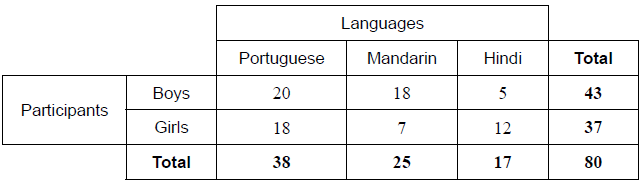A boy is chosen at random.

State the number of boys who answered questions in Portuguese.

a.

Find the probability that the boy answered questions in Hindi.

b.

Two girls are selected at random.

Calculate the probability that one girl answered questions in Mandarin and the other answered questions in Hindi.

c.

## Markscheme

20     (A1) (C1)[1 mark]

a.

$$\frac{5}{{43}}\,\,\,\left( {0.11627 \ldots ,\,\,11.6279 \ldots {\text{% }}} \right)$$     (A1)(A1) (C2)

Note: Award (A1) for correct numerator, (A1) for correct denominator.[2 marks]

b.

$$\frac{7}{{37}} \times \frac{{12}}{{36}} + \frac{{12}}{{37}} \times \frac{7}{{36}}$$     (A1)(M1)

Note: Award (A1) for first or second correct product seen, (M1) for adding their two products or for multiplying their product by two.

$$= \frac{{14}}{{111}}\,\,\left( {\,0.12612 \ldots ,\,\,12.6126\,{\text{% }}} \right)$$     (A1) (C3)[3 marks]

c.